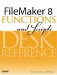# Lg()

Category: Number

 Syntax: Lg ( number )

Parameters:

numberAny expression that resolves to a positive numeric value or a field containing a numeric expression.

Data type returned: Number

Description:

Returns the base-2 logarithm of number. Negative values for number return an error.

The base-2 logarithm (often called the binary logarithm) of a number is the power of 2 that you would need to generate the number. Thus, if 2^x = y, then Lg(y) = x. The value returned by the Lg() function is increased by 1 every time that x is doubled.

Examples:

``` Lg (1) = 0

Lg (2) = 1

Lg (32) = 5
```FileMaker 8 Functions and Scripts Desk Reference
ISBN: 0789735113
EAN: 2147483647
Year: 2004
Pages: 352